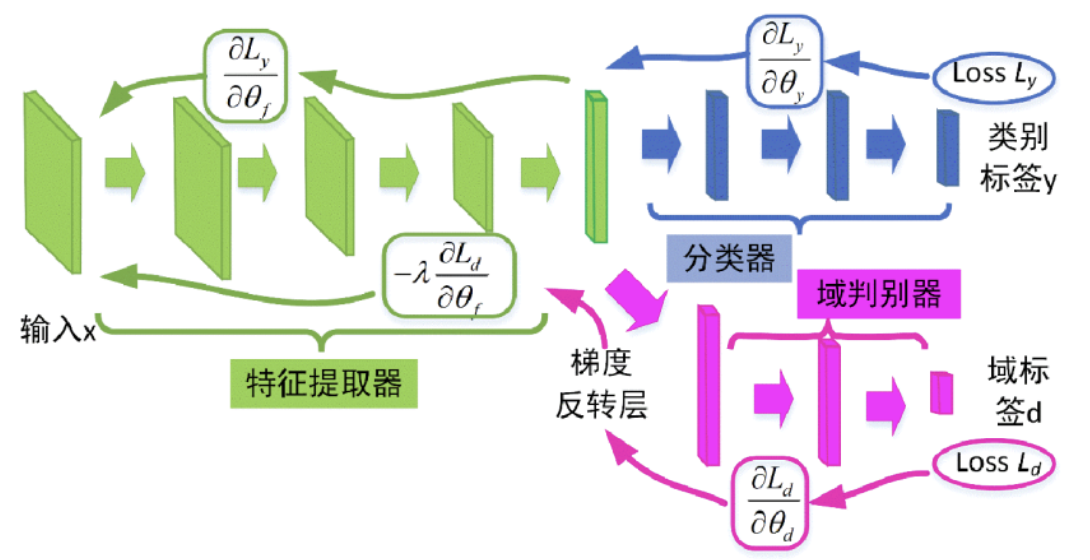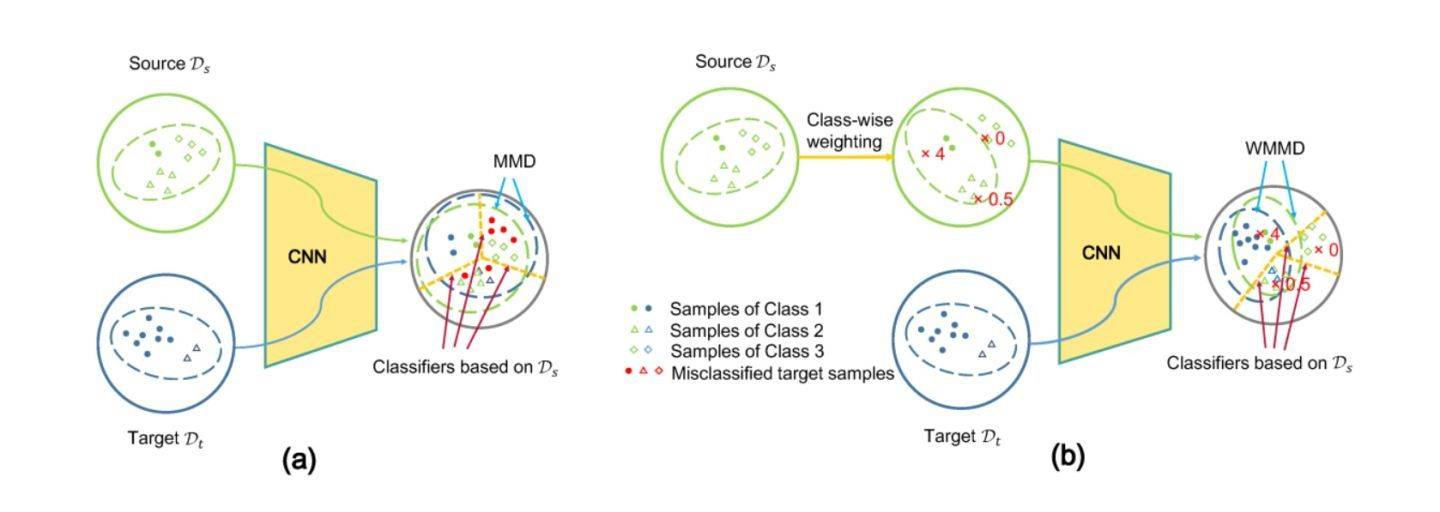# 迁移学习

• 归纳迁移学习（inductive transfer learning）：目标领域中有少量标注样本
• 直推式迁移学习（transductive transfer learning）：只有源领域中有标签样本
• 无监督迁移学习（unsupervised transfer learning）：源领域和目标领域都没有标签样本

# 域适应

• 基于领域分布差异的方法
• 基于对抗的方法
• 基于重构的方法
• 基于样本生成的方法

## 基于域对抗

Domain-Adversarial Training of Neural Networks (DANN)

### 基本结构• 特征提取器 $G_f$ (Feature Extractor)：主要负责将数据映射到对应的特征空间中。
• 分类器 $G_y$ (Classifier)：主要负责将对应特征空间的点分类输出结果。
• 判别器 $G_d$ (Discriminator)：主要负责判断该特征点来自于哪个域。

### 基本过程

1. 首先将源域和目标域的数据输入到特征提取器中得到两个特征 $F_s$ 和 $F_t$
2. 将 $F_s$ 输入到分类器后和已知标签对比计算得到分类器损失 $L_y$
3. 将 $F_s$ 和 $F_t$ 输入到判别器后和数据类型进行比较得到域判别器损失 $L_d$
4. 通过 $L_y$ 和 $L_d$ 对 特征提取器域判别器 进行优化

### 注意点

• 首先先讲清楚判别器在这里到底有啥用，为什么需要它？不妨考虑没有它的情况，没有它的话也就是一个普通的 CNN，而 CNN 难以对目标域进行准确分类，所以我们得想办法让我们的网络也能对目标域进行分类，于是我们就想到了如果同一类的目标域和源域的数据的特征接近，且源域的分类器能准确对源域进行分类，那么源域的分类器不就也可以对目标域进行分类了吗？
• 域判别器内有个梯度反转层，实现了同时的 GAN 训练和特征提取层准确率的训练，不需要像 GAN 一样需要分开训练。
• $L_y$ 和 $L_d$ 两个损失的作用：
• $L_y$ 分类器损失，在这里的作用比较明显，其实也就是让特征提取器训练的模型越来越能对 源域 能进行准确分类。$L_y$ 作用于特征提取器，让特征提取器提取出来的 源域 能进行准确分类从而在梯度下降法能提高准确率。
• $L_d$ 判别器损失，该损失是在特征空间中 $D_s$ 和 $D_t$ 的距离，在这里的作用就有两个：
1. 首先是因为存在 梯度反转层，$L_d$ 的影响本来是让特征提取器将特征 $D_s$ 和 $D_t$ 的度量增大从而能够让域判别器能更好的去判别；而加了梯度反转层后优化特征提取器，就是反的让提取出来的特征 $D_s$ 和 $D_t$ 的度量距离减小，也就是让同类的目标域特征和源域特征接近，从而实现源域分类器分类目标域。
2. 其次是去优化域判别器本身，让域判别器不断优化本身更能判断数据来自源域还是目标域，从而提高域判别准确率；就像 GAN 一样，由于上述的梯度反转层的作用让特征提取器和判别器对抗不断减小源域和目标域在特征空间中的距离，让源域和目标域在特征空间中接近从而实现源域分类器分类目标域。
• 这里可能域判别器的梯度反转层让人难以理解，我的理解是这样的：
• 首先我们考虑到如何降低在特征空间中 $D_s$ 和 $D_t$ 的距离，于是我们想到了两种方法：
• 距离计算损失
• 域判别器对抗训练（即 DANN
• 这里我们采用的是域判别器对抗训练，后面我们再说 距离计算损失 ，具体的我们通过域判别器来判定数据来自哪个域，我们假设 没有梯度反转层
• 没有梯度反转层的时候，如果我们只去 优化特征提取器 ，随着训练的不断进行梯度的不断下降，虽然确实两个域接近了，但是由于 域判别器 对于该特征的判别的权重不同，导致域判别器对其中某个域有“偏见”，导致最终训练得到的模型的源域和目标域的距离并没有我们想达到的无限接近，而是有判别器的权重影响，故该方案不可靠。
• 没有梯度反转层的时候，如果我们同时去 优化特征提取器和域分类器，采用类似于 GAN 的思想：
1. 只训练训练特征提取器让域分类器无法准确分类（准确率接近 0.5）
2. 只训练域分类器让特征提取器输出能被准确识别（准确率接近 1）
3. 重复 1 和 2 直到效果可以了即可。
• 由上述可知，当没有梯度反转的时候我们可以通过 GAN 的思想来实现源域和目标域在特征空间中接近，但是这样训练起来或许太过麻烦，于是有了我们所谓的梯度反转层来方便训练：
• 借助梯度反转层让域判别器在不断提高判别准确率的同时也让特征提取器提取出的特征空间中 $D_s$ 和 $D_t$ 的距离越来越小从而实现对抗，最终在优化器的整体作用下梯度下降，判别器本身能很好的判别了，但是由于特征提取器提取出的特征空间中 $D_s$ 和 $D_t$ 的距离越来越小导致判别器的准确率接近 0.5 了，也就是特征提取器能很好的提取出公共特征让源域和目标域能无限接近了，从而达到了 GAN 的训练目的。

## 基于度量

• MMD (Maximum Mean Discrepancy): 最大均值差异
• WD (Wasserstein Distance): 搬土距离
• CMD (Central Moment Discrepancy): 中心距差异

### 基本结构• 特征提取器 $G_f$ (Feature Extractor)：主要负责将数据映射到对应的特征空间中。
• 分类器 $G_y$ (Classifier)：主要负责将对应特征空间的点分类输出结果。

### 基本流程

1. 首先将源域和目标域的数据输入到特征提取器中得到两个特征 $F_s$ 和 $F_t$
2. 将 $F_s$ 输入到分类器后和已知标签对比计算得到分类器损失 $L_y$
3. 通过 MMD 对 $F_s$ 和 $F_t$ 计算出距离损失 $L_d$
4. 通过 $L_y$ 和 $L_d$ 对 特征提取器 进行优化

### 注意点

• 基于 MMD 本质上和 DANN 相同，都是去计算两个域的差异的损失 $L_d$ 然后再去优化特征提取器。